## Tuesday, October 11, 2011

### RPL Programming Tutorial - Part 5 - HP 49g+/50g: Prompt and Introduction to Algebraic Objects

Excuse me, but I must prompt you for something.

Welcome to the second week of my RPL tutorials, using the Hewlett Packard HP 49g+ and 50g calculators. Today's session will cover the PROMPT command.

The PROMPT command sets the program to ask the user for input. That input can be whatever you practically want: text, numbers, matrices, and equations. The user enters the requested information and presses the key sequence [LS] [ON] (CONT) . The [LS] [ON] sequence tells the calculator that the user is ready to move on.

The PROMPT structure is this:

"String that asks the user for input" PROMPT

Today we will do two programs using PROMPT: a simple program that picks an integer from 1 to N, and a more complex one that takes an equation and graphs it.

In this bag we have lotto balls from 1 to 49, and the first number is...

This program will ask you for a number (N), the number of draws (X), and the calculator will return X integers randomly selected from 1 to N. One caveat in this program is that the numbers selected can repeat.

The Program ONETO

Comments will be italicized, starting with an asterisk. You will not need to pre-load the stack with ONETO - all the inputs will be prompted for.

As a reminder:

[LS] means the left shift key (white on the 50g, green on the 49g+)
[RS] means the right shift key (orange on the 50g, red on the 49g+)
Pressing [ALPHA] twice will put the calculator in ALPHA-LOCK mode. If you want lower case letters, press [LS] then the letter. For greek and other characters, select [RS] then the letter.

Hint:

During program entry, you can press [RS] [ . ] for a carriage return. This can make program entry easier to see and read. Best of all, this does not affect program entry or execution.

Note:

The key [big X] is located on the 5th rows of keys up from the bottom. I will use this to distinguish the X character from the times key [ x ].

Program Input:

[RS] [ + ] ( << >> )
* Start the program entry
[RS] [ x ] ( "" )
[ALPHA] [ALPHA] [MODE] (H) [TOOL] (I) [APPS] (G) [MODE] (H) [SPC] [EVAL] (N) [TAN] (U) [HIST] (M) [F2] (B) [F5] (E) [ √ ] (R) [RS] [+/-] (=) [ALPHA]
[ &rarr ] [LS] [EVAL] (PRG) [NXT] [F5] (IN) [NXT] [F1] (PROMPT)

* Enters the first prompt request, "HIGH NUMBER="
[RS] [ x ] ( "" )
[ALPHA] [ALPHA] [LS] 3 (#) [SPC] [ ' ] (O) [F6] (F) [SPC] [F4] (D) [ √ ] (R) [F1] (A) [+/-] (W) [SIN] (S) [RS] [+/-] (=) [ALPHA]
[ &rarr ] [F1] (PROMPT)

* Enters the second prompt request, "# OF TIMES="
[RS] 0 ( &rarr ) [ALPHA] [EVAL] (N) [SPC] [big X]
* Enters N and X and declares them as local variables
[RS] [ + ] (<< >>) 1 [SPC] [big X]
[F6] (PRG) [F3] (BRCH) [LS] [F4] (FOR)

* Use the current menu to go back to the Program level, enters FOR-NEXT structure
[ALPHA] [STO>] (K) [SPC] [ALPHA] [EVAL] (N)
[LS] [SYMB] (MTH) [NXT] [F1] (PROB) [F4] (RAND)
[ x ] 1 [ + ] [NXT] [F6] (MTH) [F5] (REAL) [NXT] [IP] (F5)

* Enters the commands for the For loop
[ENTER]
* Terminate program entry

[ ' ] [ALPHA] [ALPHA] [ ' ] (O) [EVAL] (N) [F5] (E) [COS] (T) [ ' ] (O) [ALPHA] [ENTER] [STO>]

The completed program:

<< "HIGH NUMBER=" PROMPT
"# OF DRAWS=" PROMPT
&rarr N X
<< 1 X
FOR K N RAND * 1 + IP
NEXT >> >>

Instructions:

1. Run ONETO
2. The calculator will prompt "HIGH NUMBER=" at the top of the screen. Enter the high number and then press [LS] [ON] (CONT).
3. The calculator will prompt "# OF DRAWS=" at the top of the screen. Enter the number of draws and then press [LS] [ON] (CONT).
4. The calculator fills the stack with randomly selected numbers. Use [HIST] and [ &uarr ] to view the numbers. Press [ON] when done viewing the stack.
5. You may want to clear the stack before continuing.

Example:

A possible set of 12 random integers from 1-12 is: 9, 4, 7, 2, 11, 1, 10, 4, 1, 4, 1, 7.

Graphing an equation from the Stack Program

This program graphs a given an function y(x) using specified window settings. This is just one way of graphing an equation, which is an alternate way to graphing functions without using the graphing set up screens (Y=, WIN, GRAPH, and 2D/3D). The function can be traced and analyzed just like any other functions that are plotted.

The Catalog

You can access all the commands that the 49g+ and 50g has in the catalog. To access the catalog, press [RS] [SYMB] (CAT). If you press [ALPHA] and a letter, you can quickly scroll to that part of the catalog. You may be able to get a few letters if you are fast enough.

The other thing that is nice about the 49g+ and 50g is that the commands can be typed. This may be a helpful and faster alternate than trying to hunt commands in the menu or catalog. However, in this tutorial, the keystrokes I show will make use of the catalog.

Commands that are Used

* XRNG: Sets the left and right boundaries of the plot screen. The left boundary is taken from Level 2; the right boundary is taken from Level 1.
* YRNG: Sets the bottom and top boundaries of the plot screen. The bottom boundary is taken from Level 2; the top boundary is taken from Level 1.
* FUNCTION: Sets the calculator to Function mode.
* STEQ (Store Equation): Stores an equation in the EQ variable. The EQ variable is a system variable which the calculator uses to plot and solve equations with. EQ can have a list of more than one equation or expression.
* INDEP: Sets a variable as independent. Useful when setting up a plot. It makes sense to make the independent variable 'X' or even 'T' or ' θ', but you can make the independent variable anything you want.
* ERASE: Erases the plot screen and gets the calculator ready to draw a fresh, new plot.
* DRAW: Has the calculator draws whatever is stored in EQ.
* DRAX (Draw the Axes): Has the calculator draw the axes.
* PICTURE: Switches the calculator to the plot (picture) environment.

With all these commands to play with, let's program GRPFX.

The Program GRPFX

[RS] [ + ] (<< >>)
[RS] [ x ] ( "" )
[ALPHA] [ALPHA] [1/x] (Y) [LS] [ - ] (parenthesis) [big X] [RS] [+/-] (=)

* Input the string "Y="
[LS] [EVAL] (PRG) [NXT] [F5] (IN) [NXT] [F1] (PROMPT)
* Inserts the Prompt command. Leave this menu open.
[RS] [SYMB] (CAT) [ALPHA] [SIN] (S) scroll until you find STEQ
[F6] (OK)

* Use the catalog to find the STEQ command. You can hold [ &darr ] and [ &uarr ] to quickly scroll the catalog.
[RS] [SYMB] (CAT) [ALPHA] [F6] (F) find FUNCTION [F6] (OK)
* Set the calculator to FUNCTION mode
[ ' ] [ (big) X ] [ &rarr ] [RS] [SYMB] [ALPHA] [TOOL] (I) find INDEP [F6] (OK)
* Set X to be the independent variable.
[RS] [ x ] ( "") [ALPHA] [ALPHA] [big X] [HIST] (M) [TOOL] (I) [EVAL] (N) [RS] [+/-] (=) [F1] (PROMPT)
[RS] [ x ] ( "" ) [ALPHA] [ALPHA] [big X] [HIST] (M) [F1] (A) [big X] [RS] [+/-] (=) [F1] (PROMPT)
[RS] [SYMB] (CAT) [big X] find XRNG [F6] (OK)

* Prompt for the left and right boundaries, then use the XRNG command.
[RS] [ x ] ( "" ) [ALPHA] [ALPHA] [1/X] (Y) [HIST] (M) [TOOL] (I) [EVAL] (N) [RS] [+/-] (=) [F1] (PROMPT)
[RS] [ x ] ( "" ) [ALPHA] [ALPHA] [1/X] (Y) [HIST] (M) [F1] (A) [big X] [RS] [+/-] (=) [F1] (PROMPT)
[RS] [SYMB] (CAT) [ALPHA] [1/X] (Y) find YRNG [F6] (OK)

* Prompt for the bottom and top boundaries, then use the YRNG command.
[RS] [SYMB] (CAT) [ALPHA] [F5] (E) find ERASE [F6] (OK)
[RS] [SYMB] (CAT) [ALPHA] [F4] (D) find DRAX [F6] (OK)
[RS] [SYMB] (CAT) [ALPHA] [F4] (D) find DRAW [F6] (OK)

* Input the ERASE, DRAX, and DRAW commands.
[RS] [SYMB] (CAT) [ALPHA] [SYMB] (P) find PICTURE [F6] (OK) [ENTER]
* Input the PICTURE command and terminate program entry.

The completed program:

<< "Y(X)=" PROMPT
STEQ
FUNCTION
'X' INDEP
"XMIN=" PROMPT "XMAX=" PROMPT XRNG
"YMIN=" PROMPT "YMAX=" PROMPT YRNG
ERASE DRAX DRAW
PICTURE >>

Algebraic Objects

Simply put, algebraic objects are equations or formulas that are enclosed in single quotes. Examples include:

'1'
'X+2/5'
'SIN(π-1/2)'
'e^5.2-SQ(3)'

Algebraic objects gives the user an alternative (and maybe easier) way for the user to enter equations. In Part 6, we will explore several common functions that can be used with algebraic objects.

We can also enter equations using the RPN method. Let's try an example:

To enter 'X^2 + 3X - 1', we can enter this two ways. Assume X does not have anything stored in it. Purge X if need be.

RPN Way: [big X] [ENTER]
* duplicates X.
[LS] [ √ ] (x^2) [LS] [ &rarr ] (SWAP) 3 [ x ] [ + ] 1 [ - ] [EVAL]

Use [EVAL] to simplify the expression.

Algebraic Object: [ ' ] [big X] [y^x] 2 [ + ] 3 [ x ] [big X] [ - ] 1 [ENTER]

Instructions for GRPFX:

1. Run GRPFX. All the input will be asked for you, so you don't need anything in the stack to start with.
2. At the "Y(X)=" prompt, enter a function in X. You can use RPN, an algebraic object, or use the equation writer. Please be aware that the prompt may disappear when you are entering the function, just continue. Press [LS] [ON] (CONT) to continue.
3. At the "XMIN=" prompt, enter the left boundary, then press [LS] [ON] (CONT).
4. At the "XMAX=" prompt, enter the right boundary, then press [LS] [ON] (CONT).
5. At the "YMIN=" prompt, enter the bottom boundary, then press [LS] [ON] (CONT).
6. At the "YMAX=" prompt, enter the top boundary, then press [LS] [ON] (CONT).
7. You will see the function plotted. Use [F3] [F2] to trace the function. [F4] will give you access to function analysis such as ROOT, SLOPE, and AREA. Press [ON] to get back to the home screen.

The following pictures show GRPHX in action while plotting y(x) = x^2 + 3x - 1. Using the window, X = [-10, 10] and Y = [-10, 10]. (I am took pictures at a coffee shop using the iPad)

Hope you enjoyed Part 5. Come back for Part 6 where we explore cool functions with algebraic objects.

This tutorial is property of Edward Shore. Mass reproduction or distribution requires express permission of the author.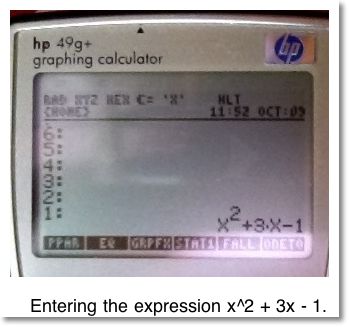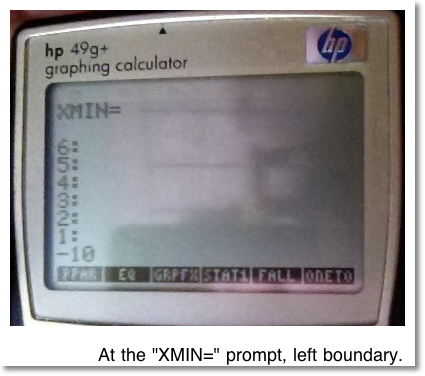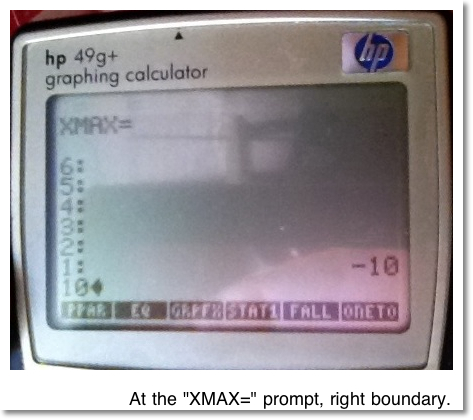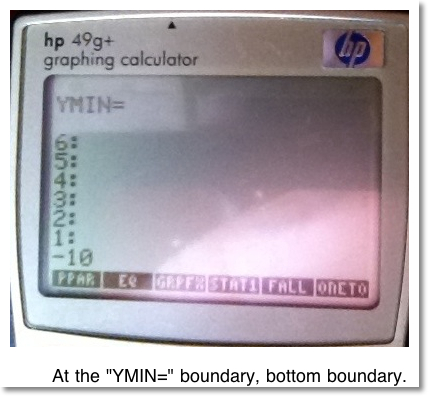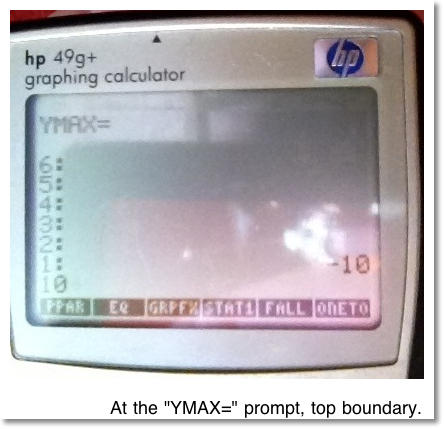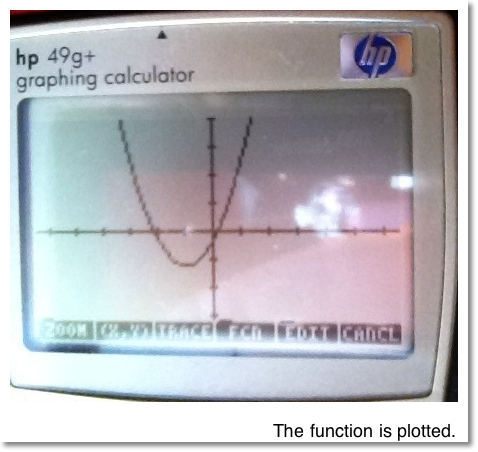### Swiss Micros DM16L: Advanced Boolean and Factorial (up to 20)

Swiss Micros DM16L:   Advanced Boolean and Factorial (up to 20) Introduction The program listing, for the Swiss Micros DM16L and Hewlett Pac...